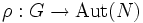# Semidirectly extensible implies linearly pushforwardable for representation over prime field

## Statement

Suppose$F$ is a prime field (i.e., either a field of prime order or the field of rational numbers), and$G$ is a group. Suppose$V$ is a finite-dimensional vector space over$F$, and$\rho:G \to GL(V)$ be a linear representation of$G$. Let$H = V \rtimes G$ with respect to the induced action of$G$ on$V$.

Suppose, further, that$\sigma$ is an automorphism of$G$ that can be extended to an automorphism$\sigma'$ of$H$ such that$\sigma'$ also restricts to an automorphism$\alpha$ of$V$. Then,$\rho \circ \sigma = c_\alpha \circ \rho$ where$c_\alpha$ is conjugation by$\alpha$ in$GL(V)$.

Note that we need the field to be a prime field in order that$GL(V)$ is equal to the automorphism group of$V$ as a group.

## Facts used

1. Automorphism group equals general linear group for vector space over prime field
2. Automorphism group action lemma: Suppose$H$ is a group, and$N,G \le H$ are subgroups such that$G \le N_H(N)$. Suppose$\sigma'$ is an automorphism of$H$ such that the restriction of$\sigma'$ to$N$ gives an automorphism$\alpha$ of$N$, and such that$\sigma'$ also restricts to an automorphism of$G$, say$\sigma$. Consider the map:$\rho: G \to \operatorname{Aut}(N)$

that sends an element$g \in G$ to the automorphism of$N$ induced by conjugation by$g$ (note that this is an automorphism since$G \le N_H(N)$). Then, we have:$\rho \circ \sigma = c_\alpha \circ \rho$

where$c_\alpha$ denotes conjugation by$\alpha$ in the group$\operatorname{Aut}(N)$.

## Proof

Given: A group$G$, a homomorphism$\rho:G \to GL(V)$ for a finite-dimensional vector space$V$ over a prime field$F$.$\sigma$ is an automorphism of$G$ that extends to an automorphism$\sigma'$ of$H$, such that$\sigma'$ also restricts to an automorphism$\alpha$ of$V$.

To prove:$\rho \circ \sigma = c_\alpha \circ \rho$.

Proof: Since$F$ is a prime field,$GL(V)$ is the whole automorphism group of$V$ by fact (1) (in general, it is a proper subgroup). Thus, the element$\alpha$, which is a group automorphism of$V$, is actually in$GL(V)$. Thus, fact (2), setting$G = G, H = H, N = V, \sigma' = \sigma', \alpha = \alpha, \sigma = \sigma$, gives the desired result.